## Selina Concise Mathematics Class 6 ICSE Solutions Chapter 7 Number Line

Selina Publishers Concise Mathematics Class 6 ICSE Solutions Chapter 7 Number Line

APlusTopper.com provides step by step solutions for Selina Concise ICSE Solutions for Class 6 Mathematics. You can download the Selina Concise Mathematics ICSE Solutions for Class 6 with Free PDF download option. Selina Publishers Concise Mathematics for Class 6 ICSE Solutions all questions are solved and explained by expert mathematic teachers as per ICSE board guidelines.

IMPORTANT POINTS

1. Number Line : A Number line is used to represent numbers, such as : fractions, whole numbers, integers, etc.
2. Using A Number Line to Compare Numbers : Out of any two numbers, marked on a number line, the number which is on the right of the other number is greater and the number which is on the left of the other number is lesser (smaller).

### Number Line Exercise 7A – Selina Concise Mathematics Class 6 ICSE Solutions

Question 1.
Fill in the blanks, using the following number line :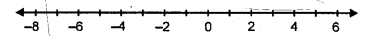(i) An integer, on the given number line, is ………… than every number on its left.
(ii) An integer, on the given number line, is greater than every number to its …………..
(iii) 2 is greater than – 4 implies 2 is to the ………….. of – 4.
(iv) -3 is ………….. than 2 and 3 is ………. than – 2.
(v) – 4 is ………….. than -8 and 4 is …………… than 8.
(vi) 5 is …………. than 2 and -5 is …………… than – 2.
(vii) -6 is …………. than 3 and the opposite of -6 is ………… than opposite of 3.
(viii) 8 is …………. than -5 and -8 is ……….. than -5.
Solution:
(i) An integer, on the given number line, is greater than every number on its left.
(ii) An integer, on the given number line, is greater than every number to its left.
(iii) 2 is greater than – 4 implies 2 is on the right of – 4.
(iv) – 3 is less than 2 and 3 is greater than -2.
(v) – 4 is greater than -8 and 4 is less than 8.
(vi) 5 is greater than 2 and – 5 is less than – 2.
(vii) -6 is less than 3 and the opposite of -6 is greater than opposite of 3.
(viii) 8 is greater than -5 and -8 is less than -5.

Question 2.
In each of the following pairs, state which integer is greater :
(i) -15, -23
(ii) -12, 15
(iii) 0, 8
(iv) 0, -3
Solution:
(i) -15, -23
-15 is greater than -23 as -15 lies on the right side of-23 on the number line
(ii) -12, 15
15 is greater than than -12 as 15 lies on the right side of -12 on the number line
(iii) 0, 8 8 > 0
(iv) 0, -3 0 > – 3

Question 3.
In each of the following pairs, which integer is smaller :
(i) o, -6
(ii) 2, -3
(iii) 15, -51
(iv) 13, 0
Solution:
(i) 0, -6
-6 < 0
(ii) 2, -3
-3 < 2
(iii) 15, -51
-51 < 15
(iv) 13, 0
0 < 13

Question 4.
In each of the following pairs, replace * with < or > to make the statement true:
(i) 3 * 0
(ii) 0 * -8
(iii) -9 * -3
(iv) 3 * 3
(v) 5 * -1
(vi) -13 * 0
(vii) -8 * -18
(viii) 516 * -316
Solution:
(i) 3 > 0
(ii) 0 > -8
(iii) -9 < -3
(iv) -3 < 3
(v) 5 > -1
(vi) -13 < 0
(vii) -8 > -18
(viii) 516 > -316

Question 5.
In each case, arrange the given integers in ascending order using a number line.
(i) – 8, 0, – 5, 5, 4, – 1
(ii) 3, – 3, 4, – 7, 0, – 6, 2
Solution:
(i) – 8, 0, – 5, 5, 4, – 1
Draw a number line and mark the numbers on it. Arranging in ascending order, as shown -8,-5,-1, 0, 4, 5 as on the number line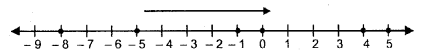(ii) 3, -3, 4, -7, 0, -6, 2
Draw the number line and mark the numbers on it. Arranging in ascending order as shown on the number line.
-7, -6, -3, 0, 2, 3, 4Question 6.
In each case, arrange the given integers in descending order using a number line.
(i) -5, -3, 8, 15, 0, -2
(ii) 12, 23, -11, 0, 7, 6
Solution:
(i) -5, -3, 8, 15, 0, -2
Draw the number line and mark these numbers on it. Arranging in descending order 15, 8, 0 -2, -3, -5 as shown on the number line(ii) 12, 23, -11, 0, 7, 6
Draw a number line and mark these numbers on it. Arranging in descending order. 23, 12, 7, 6, 0, -1 as shown on the number line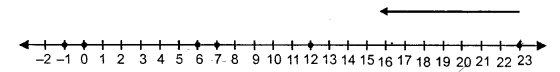Question 7.
For each of the statements, given below, state whether it is true or false :
(i) The smallest integer is 0.
(ii) The opposite of -17 is 17.
(iii) The opposite of zero is zero.
(iv) Every negative integar is smaller than 0.
(v) 0 is greater than every positive integer.
(vi) Since, zero is neither negative nor positive ; it is not an integer.
Solution:
(i) False
(ii) True
(iii) True
(iv) True
(v) False
(vi) False

### Number Line Exercise 7B – Selina Concise Mathematics Class 6 ICSE Solutions

Use a number line to evaluate each of the following :
Question 1.
(i) (+ 7) + (+ 4)
(ii) 0 + (+ 6)
(iii) (+ 5) + 0
Solution:Question 2.
(i) (-4) + (+5)
(ii) 0 + (-2)
(iii) (-1) + (-4)
Solution: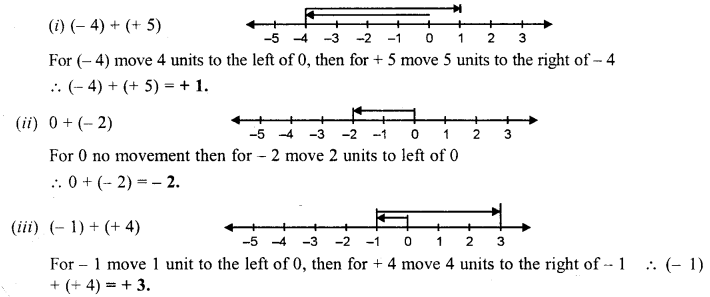Question 3.
(i) (+ 4) + (-2)
(ii) (+3) + (-6)
(iii) 3 + (-7)
Solution: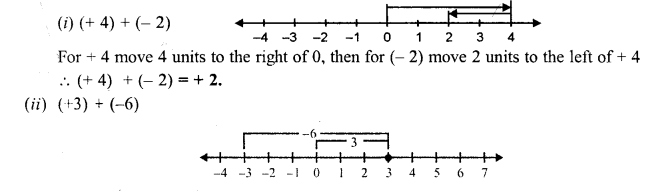Question 4.
(i) (-1) + (-2)
(ii) (-2) + (-5)
(ii) (-3) + (-4)
Solution:Question 5.
(i) (+ 10) – (+2)
(ii) (+8)- (-5)
(iii) (-6) – (+2)
(iv) (-7) – (+5)
(v) (+4) – (-2)
(vi) (-8) – (-4)
Solution: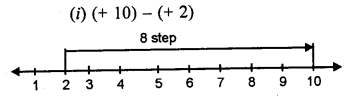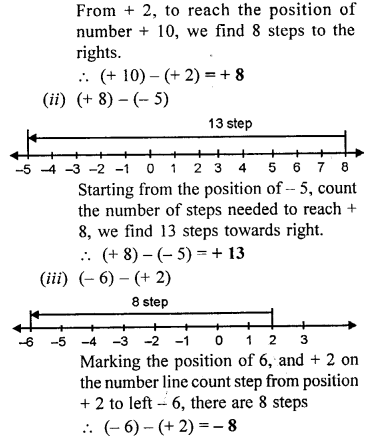Question 6.
Using a number line, find the integer which is :
(i) 3 more than -1
(ii) 5 less than 2
(iii) 5 more than -9
(iv) 4 less than -4
(v) 7 more than 0
(vi) 7 less than -8
Solution:### Number Line Revision Exercise – Selina Concise Mathematics Class 6 ICSE Solutions

Question 1.
Fill in the blanks :
(i) 5 is …………… than -2 and -5 is ………… than 2.
(ii) -3 is ………… than 0 and 3 is …………. than 0.
(iii) on a number line, if x is to the left of y, then x is ………… than y.
(iv) on a number line if x is to the right of y, then y is …………. than x.
Solution:
(i) 5 is greater than -2 and -5 is less than 2.
(ii) -3 is less than 0 and 3 is greater than 0.
(iii) On a number line, if x is to the left of y, then x is less than y.
(iv) On a number line, x is to the right of y, then y is less than x.

Question 2.
Using a number line, write the numbers -15, 7, 0, -8 and -3 in ascending order of value.
Solution:
On the given number line, we mark the numbers -15, 7, 0, -8 and -3 on it, we see thatWe see that -15 < -8 < -3 < 0 < 7
-15, -8, -3, 0, 7 are in ascending order

Question 3.
Using a number line, write the numbers 8, -6, 2 -12, 0, 15 and -1 in descending order of value.
Solution:
On the given number line, we mark the numbers 8, -6, 2, -12, 0, 15 and -1 on it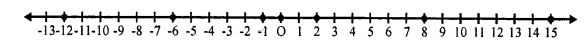We see that
15 > 8 > 2 > 0 > -1 > -6 > -12
15, 8, 2, 0, -1, -6, -12 are in descending order

Question 4.
Using a number line, evaluate :
(i) (+5) + (+4)
(ii) (+6) + (+8)
(iii) (-3) + (+5)
(iv) (-3) + (+7)
(v) (+6) + (-2)
(vi) (-3) + (+3)
(vii) (-5) + (-5)
(viii) (-7) + (-1)
(ix) (+6) – (+2)
(x) (+5) – (-3)
(xi) (+4) – (-1)
(xii) (-7) – (-2)
Solution:
(i) (+5) + (+4)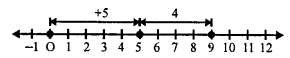First of all, we move 5 units to the right of zero then for (+4), move 4 units right of 5, then we reach at 9, then
(+5) + (+4) = +9
(ii) (+6) + (+8)First of all, we move 6 units to the right of zero then for (+8), we move 8 units to the right of (+6)
Then we reach at +14, then
(+6) + (+8) = +14
(iii) (-3) + (+5)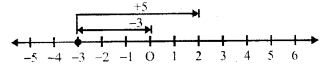First of all for (-3) we move, 3 units to the left of zero, then move (+5) units to the right of 5, then we reach at (+2), then
(-3) + (+5) = -3 + 5 = 2
(iv) (-3) + (+7)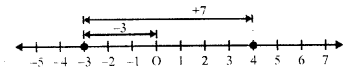First of all, we move for (-3) 3 unit to the left of zero and then for (+7), we move 7 units to the right of (-3) reaching +4 Then (-3) + (+7) = +4
(v) (+6) + (-2)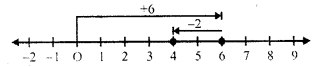First of all, we move for (+6), 6 units to the right of zero and then for (-2), move 2 units to the left of 6, then we reach 4 Then (+6) + (-2) = 6 – 2 = 4
(vi) (-3) + (+3)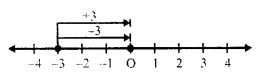First of all for (-3), we move 3 units left of zero and then for (+3) we move 3 unit right of (-3) reaching at 0
So, (-3) + (+3) = 0
(vii) (-5) + (-5)First of all for -5, we move 5 units to left of zero and then for (-5), we move 5 units to left of (-5) reaching at -10
(-5) +(-5) = -10
(viii) (-7) + (-1)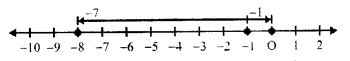First of all for -7, we move 7 units left of zero and then for (-1) we move 1 unit left of -7 reaching -8
(-7) + (-1) = -8
(ix) (+6) – (+2)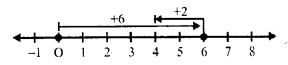First of all for (+6) we move 6 units right of 0 and then for (+2), we move 2 units left of 6 reaching 4
(+6)-(+2) = 6 – 2 = 4
(x) (+5) – (-3)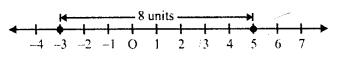Mark the points (+5) and (-3) on the same number line. We see that the position of (-3) is 8 units from (+5) to its right 3.
(+5) – (-3) = 5 + 3 = 8
(xi) (+4) – (-1)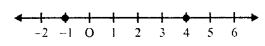Mark the points (+4) and (-1) on the same number line, we see that the position of (-1) is 5 units from (+4) to its right
(+4) – (-1) = 4 + 1 = 5
(xii) (-7) – (-2)Mark the points (-7) and (-2) on the same number line, we see that (-2) is 5 units on the left (-2)
-7 – (-2) = -7 + 2 = -5